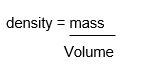SherpaQuestion

# How would you describe the typical density of a gas?

1 year ago

·

3 Replies

·

1262 views

N

Nicolette MrazNeed help with your studies? Find a qualified and experienced tutor on Sherpa.

H
AAA

Gases have very low density compared to liquids and solids. Let’s look at why.

Density is a measure of how packed the particles are in any given material/substance/thing . Density is defined as mass( mass is measure of how many particles there are) per unit volume(a unit volume means one unit of volume such as 1cm3, 1m3, 1 dm3, 1 mm3 etc). In a gas the particles are spread apart the most, compared to liquids and solids. Therefore, in a given unit of volume there will be lesser number of particles in a gas compared to the number particles in the same volume of liquid and solid. This makes the gases less dense. Let’s try to understand in the context of the density formula as well.The formular for working out the density of a substance:For example, if we consider taking 1cm3 of a gas, liquid and a solid, the mass (the top number) is lowest for the gas. This is because the particles are far apart in a gas. If the particles are spread apart a lot then there will fewer number of particles making the mass very low of the gas.  In this example we know that we are taking 1cm3 of each, so the bottom number remains the same. What changes is the top number for the three states. Smaller the top number, smaller the density value, which means less dense the gas is.

This is why you can lift a big inflated beach ball very easily than a small brick, it's the same reason why a beach ball would float and the small brick would sink if you throw them both to the water.Verified Sherpa Tutor

Of all the common states of matter, gases have the lowest densities because their atoms or molecules are furthest apart from one another with typically no or hardly any interaction. Typically then you could expect a metre cubed of gas to weigh about 1 kilogram i.e the density is about 1kg/m^3.

A very experienced teacher with a natural empathy for students

Interested in booking a 1-1 lesson with me?Verified Sherpa Tutor

Typically the density of gas is very low. There are very few particles per unit of volume because particles are randomly arrnaged and spaced out. Remeber density refers the number of particles per unit volume.

UCL Medical student with 3 years of tutoring experience

Interested in booking a 1-1 lesson with me?